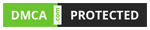Technology

# How to Find the Area of Different Types of Triangles?

Students usually learn about triangles in their mathematics classes, including geometry and trigonometry. By learning everything about the types, area, and perimeter of a triangle, students will gain an understanding of this important shape and its applications in everyday life. Generally, the area of a triangle is calculated using formula ½ × Base × Height where “Base” is any side of the triangle and “Height” is the length of the altitude drawn to the “Base” from its opposite vertex. But how to find the area in cases when we don’t have the altitude of any side or when the length of its three sides is given. The area of triangle with 3 sides and some other formulas are used in such cases to calculate the area of a triangle. Let’s learn about types of triangles and ways to calculate their area.

## Types of Triangles

A triangle is a two-dimensional shape that has three sides and three vertices. The sum of all the angles of a triangle is 180 degrees. The triangles are further classified into different types based on the length of their sides and angels. There are mainly three types of triangles:- Equilateral Triangle, Isosceles Triangle, and Scalene Triangles.

An equilateral triangle is a triangle that has all the sides equal in length. The angle formed at each vertice of such a triangle is 60 degrees. The isosceles triangle is a triangle that has two equal sides and two equal angles. A scalene triangle is the most common type of triangle; it has no equal sides and no equal angles.

## Formula to Calculate Area of Triangle

Calculating the area of a triangle is an important math skill used by many professionals in different fields.

The generic formula applied to find the area of a triangle is 0.5 * base * height, where ‘base’ represents the length of the base of the triangle, and height ‘represents’ the height/altitude of the triangle.

However, in certain cases, it’s difficult to find the height of the triangle. In these situations, many other equations can be applied, depending on the given values of the triangle:

## Area of Triangle With 3 Sides Formula

If you have the lengths of all three sides, use the Heron’s formula to calculate the area of a triangle. Heron’s formula is applied to find the area of a triangle with three sides which says if a, b, and c are the three sides of a triangle, then its area is,

Area = √[s(s-a)(s-b)(s-c)]

Here, “s” is the semi-perimeter of the triangle. i.e., s = (a + b + c)/2. But there are 3 alternate formulas to find the same.

Area = 0.25 * √( (a + b + c) * (-a + b + c) * (a – b + c) * (a + b – c) )

## Area of Triangle With 2 Sides and Angle Between Them

If the length of the two sides and the angle between them is known, you can use the following formula to calculate the area of a triangle easily:

Area = 0.5 * a * b * sin(γ)

## Area of Triangle With 2 Angle and Side Between Them

There are different versions of the area of triangle formulas that can be used to calculate the area. For example, trigonometry or law of sines to derive it:

area = = a² * sin(β) * sin(γ) / (2 * sin(β + γ))

## Area of an Equilateral Triangle

The total space enclosed within the sides of an equilateral triangle is called the area of an equilateral triangle. It is calculated by using the formula √3/4 × (side)2.

For example, if the length of a side of an equilateral triangle is 2 cm, then the area of this triangle can be √3/4 × (2)2 = √3 sq. centimeter.

### Conclusion

Triangle is an important geometric shape vastly applied in fields like designing, graphics, architecture, construction, etc. It is helpful to relate to various designs and to build structures and artworks. It is crucial for students to have knowledge about triangles, their properties, and dimensions as it enables them to understand their applications. Cuemath offers interactive math resources for students to efficiently learn an in-depth understanding of these concepts easily. To find some of the best math learning resources, visit website and learn more.

Computer

Apple

## How To Pair Apple Watch To iPhone & Macbook